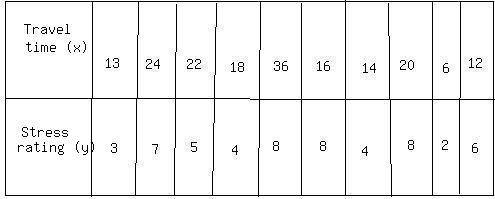Ask question

# A water tower can hold 52,000 liters of water. It currently contains 13,000 liters and is being filled with 90 liters per minute. How many hours are remaining for it to get filled?# A water tower can hold 52,000 liters of water. It currently contains 13,000 liters and is being filled with 90 liters per minute. How many hours are remaining for it to get filled?

Question
Measurementasked 2021-02-10
A water tower can hold 52,000 liters of water. It currently contains 13,000 liters and is being filled with 90 liters per minute. How many hours are remaining for it to get filled?

## Answers (1)2021-02-11
First, find out how many liters are yet to be filled:
52,000-13,000=39,000
To find out how much time is left, divide the amount yet to be filled by the rate, which is given:
39,000/90 $$\displaystyle\approx$$ 433.33 minutes
Convert to hours by dividing by 60:
433.33/60=7,22 hours
It will take 7,22 hours for the water tower to get filled.

### Relevant Questionsasked 2021-02-21
Marty asked some of his classmates to rate their level ofstress out of 10, with 10 being very high. He also asked them tomeasure th enumber of minutes it took them to get from home toschool. A random selection of his results is listed below.A.) Explain what a positive value for thecoefficient of correlation indicates.asked 2021-03-29
Two stationary point charges +3 nC and + 2nC are separated bya distance of 50cm. An electron is released from rest at a pointmidway between the two charges and moves along the line connectingthe two charges. What is the speed of the electron when it is 10cmfrom +3nC charge?
Besides the hints I'd like to ask you to give me numericalsolution so I can verify my answer later on. It would be nice ifyou could write it out, but a numerical anser would be fine alongwith the hint how to get there.asked 2021-04-25
A log 10 m long is cut at 1 meter intervals and itscross-sectional areas A (at a distance x from theend of the log) are listed in the table. Use the Midpoint Rule withn = 5 to estimate the volume of the log. (in $$\displaystyle{m}^{{{3}}}$$ )Show transcribed image text A log 10 m long is cut at 1 meter intervals and itscross-sectional areas A (at a distance x from theend of the log) are listed in the table. Use the Midpoint Rule withn = 5 to estimate the volume of the log. (in $$\displaystyle{m}^{{{3}}}$$)asked 2021-03-30
A solenoid is wound with a single layer of insulated copperwire of diameter 2.500 mm and has a diameter of 5.000cm and is 1.800 m long. Assume that adjacent wirestouch and that insulation thickness is negligible.
a)How many turns are on the solenoid?
b)What is the inductance per meter (H/m) for thesolenoid near its center?asked 2021-05-13
A rectangular barge, 5 m long and 2 m wide, floats in freshwater.
a. Find how much deeper it floatswhen its load is a 400 kg horse.
b. If the barge can only be pushed15 cm deeper into the water before water overflows tosink it, how many 400 kg horses can it carry?asked 2021-03-15
A copper calorimeter can with mass 0.100 kg contains 0.160 kgof water and 0.018 kg of ice in thermal equilibrium at atmospheric pressure.
If 0.750 kg of lead at a temperature is dropped into the calorimeter can, what is thefinal temperature? Assume that no heat is lost to the surroundings.asked 2021-05-18
The student engineer of a campus radio station wishes to verify the effectivencess of the lightning rod on the antenna mast. The unknown resistance $$\displaystyle{R}_{{x}}$$ is between points C and E. Point E is a "true ground", but is inaccessible for direct measurement because the stratum in which it is located is several meters below Earth's surface. Two identical rods are driven into the ground at A and B, introducing an unknown resistance $$\displaystyle{R}_{{y}}$$. The procedure for finding the unknown resistance $$\displaystyle{R}_{{x}}$$ is as follows. Measure resistance $$\displaystyle{R}_{{1}}$$ between points A and B. Then connect A and B with a heavy conducting wire and measure resistance $$\displaystyle{R}_{{2}}$$ between points A and C.Derive a formula for $$\displaystyle{R}_{{x}}$$ in terms of the observable resistances $$\displaystyle{R}_{{1}}$$ and $$\displaystyle{R}_{{2}}$$. A satisfactory ground resistance would be $$\displaystyle{R}_{{x}}{<}{2.0}$$ Ohms. Is the grounding of the station adequate if measurments give $$\displaystyle{R}_{{1}}={13}{O}{h}{m}{s}$$ and R_2=6.0 Ohms?asked 2021-04-21
The best laboratory vacuum has a pressure of about $$\displaystyle{1.00}\cdot{10}^{{-{18}}}$$ atm, or $$\displaystyle{1.01}\cdot{10}^{{-{13}}}{P}{a}$$. How many gas molecules are there per cubic centimeter in such a vacuum at 293 K?asked 2021-02-14
Mg reacts with H+ (aq) according to
$$\displaystyle{M}{g{{\left({s}\right)}}}+{2}{H}+{\left({a}{q}\right)}\to{M}{g}_{{2}}+{\left({a}{q}\right)}+{H}_{{2}}{\left({g}\right)}$$
Suppose that 0.524 g of Mg is reacted with 60.o ml of 1.0 M H+(aq). Assume that the density of the H+ (aq) solution os 1.00 g/ml,and that its specific heat capacity equals that of water. Theinitial and final temperatures are 22.0 degree celsius and 65.8degree celsius.
a) Is the reaction endothermic or exothermic?
b) Calculate $$\displaystyle\triangle{H}$$ of of th reaction. Use correct sigs andgive units.
c) Calculate the $$\displaystyle\triangle{H}$$ of the reaction per mole of magnesium.asked 2021-02-19
How many turns of wire would be required to make a 130-mHinductance out of a 30.0-cm-long air-filled coil with a diameter of5.2 cm?
...